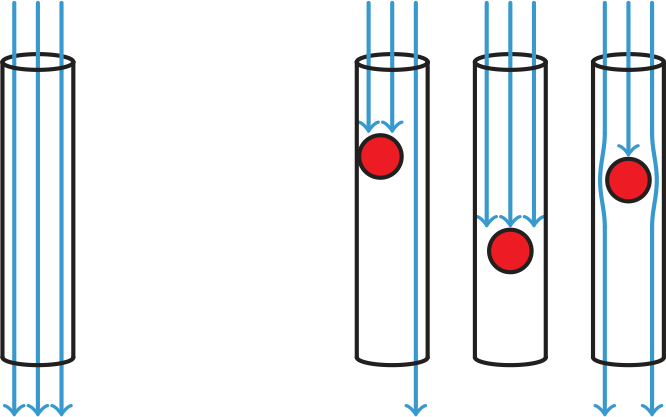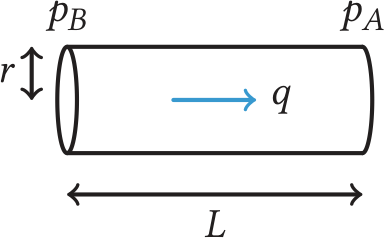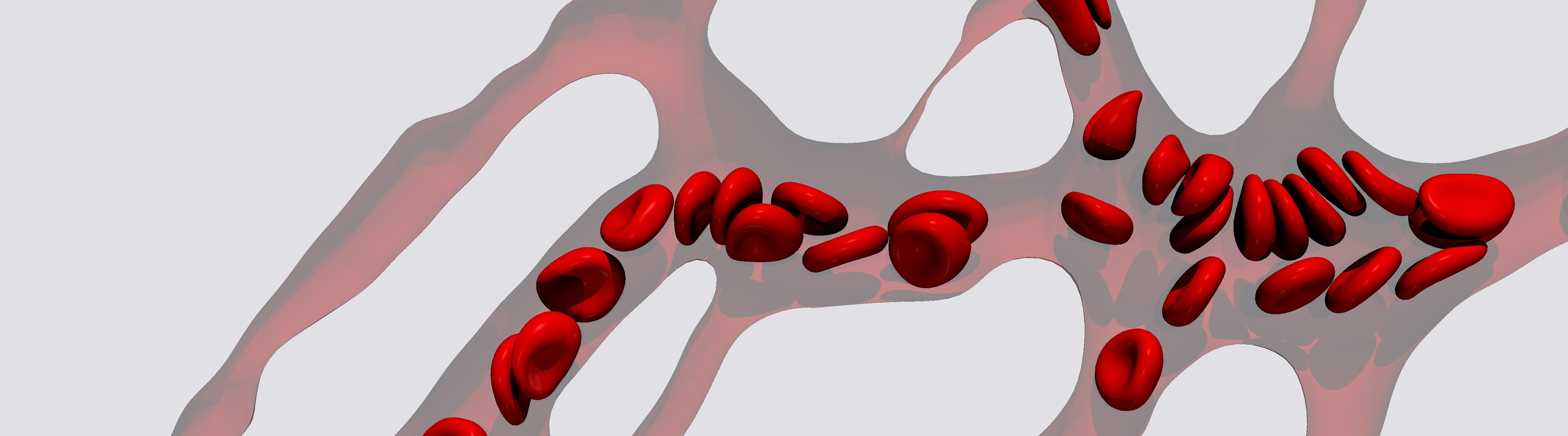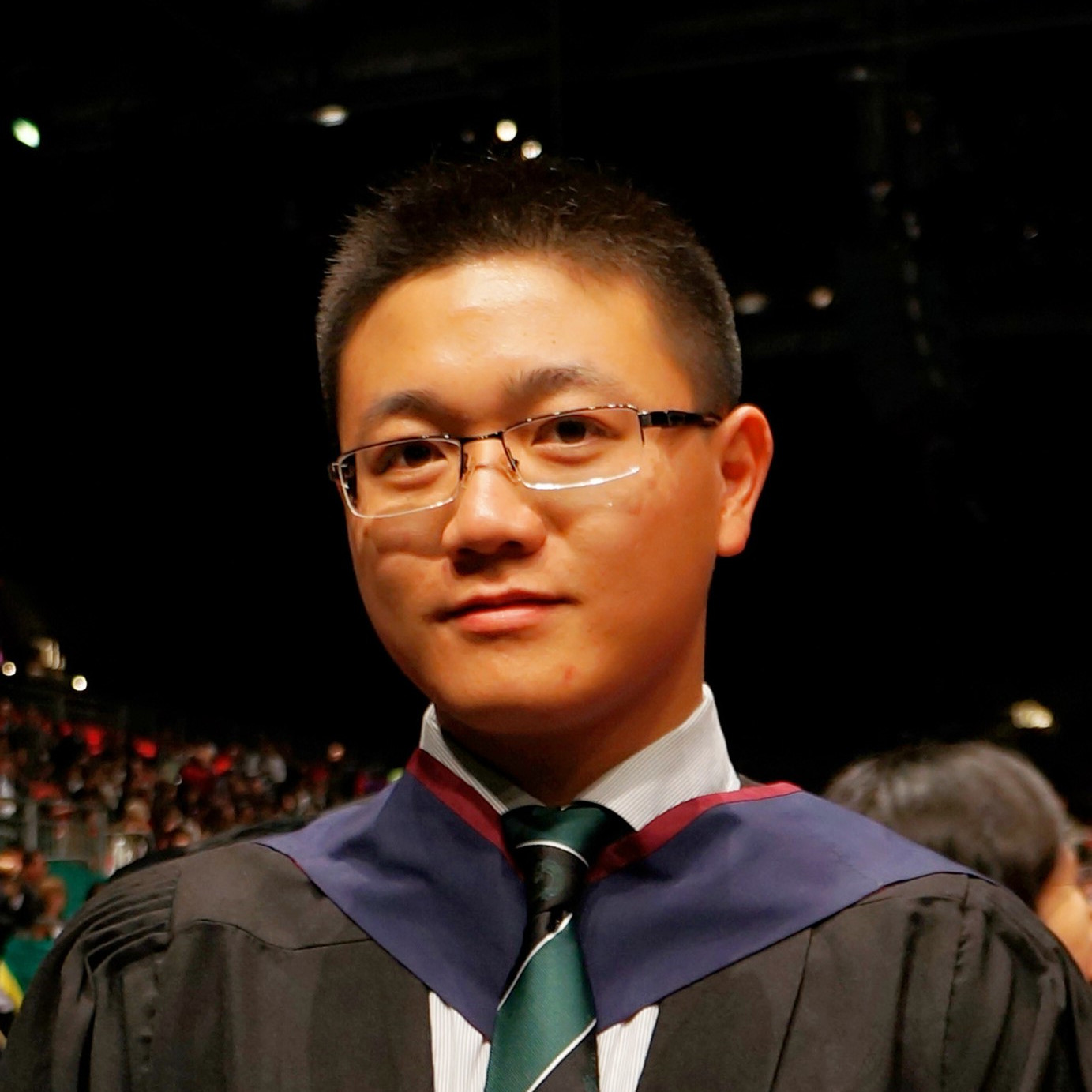# Mathematics and pregnancy

Eleanor Doman and Qi Zhou swap percentages for placenta-gesMathematics has many practical applications throughout our everyday lives, but people always seem to be surprised by its relevancy to biology and medicine. Decades, if not centuries, of work in different areas of applied mathematics have allowed us to understand how fluid flows in different situations and how materials deform under varying loads. We are part of a growing interdisciplinary research field interested in how we can apply this mechanistic understanding to the processes in the human body to better understand what goes wrong in the case of diseases and illnesses, and how we can improve the situation using interventions such as surgery or medication.

In particular we work on understanding the placenta, an organ which develops during pregnancy to supply a baby with oxygen and nutrients. Pregnancy, and the various aspects associated with it, have long been problematic to research. The standard medical research routes, such as clinical trials, are rife with ethical and practical considerations when pregnancy is involved due to concerns around the health of the foetus and mother. Both the placenta and the foetus dramatically change throughout the course of pregnancy, and our understanding of the development of both is limited. Although pregnancy can be studied in animals, the human placenta and foetus are fundamentally different to those in rabbits and rats, the animals most used in testing.

So how can we proceed? There are a number of research groups globally working on developing new techniques to investigate pregnancy. We are going to give you a taster of the mathematics that our research network is using to develop theoretical models to better understand the human placenta.

## What is the placenta?

The placenta is an organ which develops during pregnancy alongside the foetus within the uterus or womb. It enables nutrients and gases such as oxygen to be transferred from the mother’s blood into the blood supply of the foetus throughout pregnancy. Once the baby takes their first breath, the placenta is no longer required and is expelled from the mother’s body as part of the afterbirth. The placenta shares both the mother’s and the baby’s DNA, and if you’ve ever wondered about how a mother and a baby can have different blood groups—it’s down to the fact the mother’s and baby’s blood circulations are distinct flow systems within the placenta.

Within the placenta, the mother’s and foetus’s blood come close enough to allow the transfer of vital nutrients across a membrane, but do not come into contact. The foetal blood flows into and out of the placenta via the umbilical cord. Once within the placenta, the foetal blood flows through a series of increasingly small capillaries within tissue protrusions known as villi. Maternal blood is channelled from the walls of the uterus into the placenta before flowing through the spaces formed between the villi, known as the intervillous space, and exiting the placenta through veins in the base of the placenta. If the flow conditions within the intervillous space impairs the transfer of nutrients and gases into the foetal blood flow then this can potentially harm the foetus.

Understanding blood flow within the placenta is easier said than done. Experiments using placentas, either during pregnancy or after birth, are difficult to carry out due to ethical considerations and the fact that placentas can be damaged during birth. Every placenta, like every baby and woman, is unique and the exact content of blood varies from person to person. However, imaging techniques are improving and it is now possible to reconstruct 3D images of what the microscale blood vessels in a placenta may look like using placentas obtained after birth. With these 3D images, we have a better prospect than ever before of being able to computationally simulate blood flow in the placenta.

## Mathematics and blood flow

Blood is a difficult fluid to model, in part due to its complex composition. Blood contains both a fluid component, known as blood plasma, and a variety of different cells, the most common of which are red blood cells which carry oxygen around the body. The blood plasma makes up over half of the volume of blood and is over 90% water, with the other 10% being solutes such as sugars, salts, and proteins. So it’s fair to say that the majority of blood is water. Water and other similar fluids have been widely studied in a variety of different applications of mathematics, including meteorology, aeronautics, and many other industrial applications. We have a plethora of mathematical tools at our disposal when thinking about fluids such as water. Can’t we just use these tools? Well… it depends.

Let’s think about an example to illustrate the problem. If we take a tank of water and a tank of water with plastic beads in, we’re not going to see a fundamental difference in the flow behaviour between these two tanks, assuming the plastic beads are evenly dispersed throughout the water. Similarly if we pour both tanks into large drainage pipes, we also will not see any marked differences in the flows:However, if we were to pour both fluids into pipes with diameters closer in size to the beads, then there would be differences. The water with beads will not flow the same way as the water alone—the beads may partially or fully block the vessels, and at the very least we expect a reduction in flow:There’s an equivalent problem with modelling blood. Red blood cells have a diameter of approximately 8μm. The largest blood vessel in the human body is the aorta with a diameter of about 3 cm. Although there will be small differences in the flow of blood through an aorta versus the flow of plasma or a pure fluid, these differences will be minor compared to the effects of variation between individual aortas and other sources of error introduced in the experimental process. In the case of these large vessels, modelling blood using the same approaches as for fluids such as water is a fair assumption. However, in the placenta blood vessels can have diameters down to approximately 6μm. At this scale we need different approaches to those we would use with fluids such as water.

So what can we do? One property we know any fluid, including blood, must satisfy is that of mass conservation. This is essentially that a flow must obey the rule `what goes in, must come out’. If we tip one litre of fluid into an empty bucket, then we know there will be one litre of fluid in that bucket. Similarly, if we empty a bucket into a measuring jug and find that we have one litre of fluid, we know that the bucket must have initially contained one litre of fluid.

We can apply the same argument to flow through a vessel or a pipe. However, we must take into account the velocity of the fluid in and out of the vessel, and the size of the vessel. We do this by introducing the notion of volume flux, often denoted using $q$. Volume flux is a way of quantifying fluid flow and is defined as the rate of volume flow across a given area, in our case the cross-section of a vessel. If we take the volume of fluid in a vessel to be $V$, then the rate of change of $V$ in a vessel must be equal to the difference in the volume flux in and out of that vessel:This can be written as
$\frac{\mathrm{d}V}{\mathrm{d}t}=q_\text{in}-q_\text{out}.$
We can extend this concept to more complex geometries. In particular, mass conservation is useful when considering the junctions between vessels. Let’s take the example of a network consisting of one larger vessel splitting into two smaller vessels:If we again take $V$ to be the total fluid within this network, the rate of change of fluid within these vessels is given by
$\frac{\mathrm{d}V}{\mathrm{d}t}=q_\text{in}-q_a-q_b.$
Note that $V$ is an arbitrary volume based on the length scale of the network which we can label as $\mathrm{d} x$. If we take the limit $\mathrm{d} x \to 0$, so our network is reduced to flow in and out of a single point at the centre of the junction, we see that $V$ becomes infinitesimally small, allowing us to reduce our equation to
$q_\text{in}=q_a + q_b. \tag{1}$
This tells us what the flow is around a junction between vessels. But what about the flow within a single vessel? The most common method to evaluate fluid flow is using the Navier–Stokes equations, the series of 3D partial differential equations for fluid motion, given the density and viscosity of the fluid. The existence and smoothness of solutions to the Navier–Stokes equations is one of the seven millennium prize problems and is currently unsolved. However, we do have solutions to the Navier–Stokes equations in certain physical situations. One of these is the Hagen–Poiseuille equation which states that the pressure change, $p_B-p_A$, over a long cylindrical pipe is proportional to the rate of change of volume of fluid passing through the pipe. This is often stated as
$p_B-p_A = \frac{8 \mu L q}{\pi r^4}, \tag{2}$
where $r$ is the radius of the pipe, $\mu$ is the viscosity of the fluid, and $L$ is the length of the pipe:The Hagen–Poiseuille equation provides a good approximation for the flow of many fluids in pipes, which can be seen as analogous to blood vessels. But both the Navier–Stokes equations and the Hagen–Poiseuille equation make the assumption that the viscosity of the fluid, $μ$, or the friction between molecules within the fluid, is constant. This is known as a Newtonian fluid, and examples include water and air. Due to the composition of blood, the viscosity is not constant and past work has shown the viscosity of blood is a function of the proportion of red blood cells in the flow and the size of the vessels. As blood is a non-Newtonian fluid, we cannot use the Hagen–Poiseuille equation to describe the blood flow, but we can use it to inform our approach: we can take $μ$ to be dependent on parameters such as the size of the vessel and the amount of red blood cells in the vessel and still use equations of the same form as the Hagen–Poiseuille equation with variable viscosity. This is not a perfect solution to our modelling problem and would not be guaranteed to give the absolute values for the flow properties in all possible cases, but it allows us to build a model able to investigate complex blood flow.

Equation (1) describes the volume flux of blood between vessels and equation (2) tells us what we need to determine the flux through each vessel. By combining both these equations in a network of vessels, we can determine the flux through the network, given the flow in and out of the network. However for biological tissues such as the placenta, this can result in keeping track of many hundreds and thousands of vessels and junctions between vessels. As mathematicians we tackle this by taking inspiration from network theory. We define a matrix, $\boldsymbol{\mathsf{A}}$, known as the incidence matrix, which describes the relationship between each vessel and each junction. Each element of $\boldsymbol{\mathsf{A}}$ is defined as

$A_{ij} = \begin{cases} 1 & \text{if vessel j flows into junction i,} \\ -1 & \text{if vessel j flows out of junction i,} \\ 0 & \text{otherwise.}\end{cases}$

Using the incidence matrix notation we can reduce the hundreds of copies of the equations to a series of matrix equations which are solved using numerical methods within programming software such as Matlab or Python. Work on how to describe blood viscosity and other flow properties required as input for this model, in terms of the cell content of blood and vessel geometry, has been going on since the 1980s. However, this work has not been specific to the placenta: vessels in different organs will have different geometric properties, and there are still many open problems related to describing blood flow in the placenta.Simulation of cellular blood flow modelled as a suspension of red blood cells in plasma across a microvascular network during development

## Mathematics and…

We are of the opinion that the best mathematical modelling is never done in isolation. Some of the most impactful research in recent years has come about from mathematicians collaborating with researchers in other disciplines. The equations we’ve looked at and other equations in fluid dynamics can give us an insight into the function of the placenta but only with input from other disciplines to estimate the missing parameters such as viscosity and vessel geometry.

If you think about the number of babies that are born every day, there are a lot of placentas being disposed of! There are a lot of different measurements we could obtain from these placentas which could help with mathematical modelling. Unfortunately, placentas can become damaged during birth, so not every one donated to science is usable, and relatively few maternity wards are attached to research centres so most don’t have the facilities to conduct experiments with placentas. So, despite the fact that human placentas should be easier to obtain than, for example, human hearts or lungs, there is still a disparity in the amount of direct medical and biological research being done with the placenta compared to other organs.

Other ways exist to get valuable information to improve our mathematical modelling. One method is to reconstruct realistic microscale blood vascular geometries using plastics or polymers, allowing us to vary the composition of the fluid and obtain measurements of volume flux against which we can parametrise our mathematical model. This is known as microfluidics. Microfluidic chips can be constructed—for example by using 3D printing or by milling vessels within solid sections of plastic—using information on the vessel geometry obtained from imaging sections of organs. We are then able to flow solutions through these microscale geometries to understand the flow within the microscale blood vessels in our body. We say solutions here, simply because this approach gives us a large degree of freedom in terms of what fluid is used. Water and glycerol combinations can be used to consider Newtonian flow with different densities and viscosities. Real blood can also be used, but this comes with problems in terms of the cell variation between samples and length of time each sample can be used for. The use of artificial capsules and droplets shows promise by offering control of the flow properties to experimentalists and allowing us to replicate blood properties.

We can further explore recreating blood vessel geometries to inform mathematical modelling using high performance computing. Here we can use methodologies such as finite element, lattice Boltzmann and immersed boundary methods to simulate cell interactions on the microscale level within the vessels. These methods are highly computationally expensive and require specialist experience to use when considering non-Newtonian fluids (eg blood) within complex blood vessel geometries (eg the placenta); but they can provide a large amount of information for mathematical modellers and, as in the case of microfluidics, a great deal of control over the experimental system.

There is still a great deal of work to be done to advance our understanding of the placenta, and by extension pregnancy and women’s health. In 2021, the UK government published a report recognising that women receive poorer healthcare than men. There are many reasons as to why this could be: prevalence of different diseases, access to healthcare or even implicit bias. But one reason is consistent in the many reviews on gender bias in medicine: we simply know less about female-assigned bodies compared to male-assigned bodies. There are many open problems in medicine which need to be addressed to counter gender and minority bias in medicine.

One of mathematics’s greatest strengths is its ability to investigate problems involving many parameters and variables with minimal financial and time costs, and this is increasingly becoming an asset to medical research. We believe that mathematical modelling will have a crucial role in advancing medicine in the coming decades and we hope we’ve convinced you that placental research is an exciting area to be in.Eleanor is a postdoctoral researcher at the University of Manchester. A mathematician by training, she works on developing mathematical models to improve our understanding of biological mechanisms in medicine, with particular interests in women’s health and autoimmune conditions. When not doing mathematics, she crochets, sews and reads everything and anything.Qi is a research associate working on blood flow modelling in human placenta with the BioFM group at the school of engineering, University of Edinburgh. His current research focuses on the fluid dynamics and solute transport in vascular networks and biological tissues, using lattice Boltzmann and immersed boundary methods.